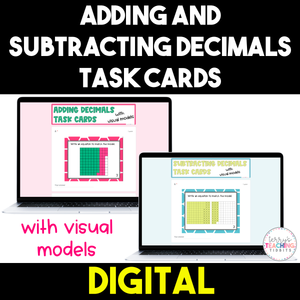• \$2.00
Unit price per
Shipping calculated at checkout.

Have your students digitally practice adding and subtracting decimals with visual models and the standard algorithm with these sets of Google Forms.

There are 12 questions in each form.

Types of questions in each form:
• a visual model is provided and students create the matching equation
• an equation is provided and students will use the standard algorithm to solve
• word problems are given and students will use the standard algorithm to solve

Standard: 5.NBT.7